### Numerical On Over-current Relay

2.16 Numerical on Over-current relay

1. Determine the time of operation of a 5 ampere, 3 second over-current relay having a current setting of 125 % and a time setting multiplier of 0.6 connected to supply circuit through a 400 / 5 current transformer when the circuit carries a fault current of 4000 amp. Use the curve shown in figure 2.8.

Solution: - Rated secondary current of CT = 5 amp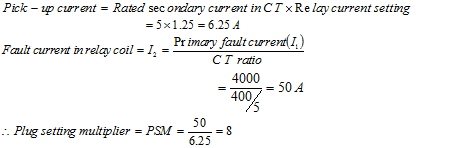From figure 2.8, corresponding to the plug setting multiplier of 8, the time of operation is 3.5 seconds.

Now, for TSM = 0.6 -

Therefore,

Actual relay operating time    = Time of operation x Time setting multiplier

= 3.5 x 0.6 = 2.1 seconds

2.     An over-current relay having a current setting of 125 % is connected to a supply circuit through a current transformer of ratio 400 / 5. Find the pick-up value.

Solution: -

Pick-up current     = Rated secondary current in CT x Relay current setting

= 5 x 1.25 = 6.25 A

3. The pick-up value of a relay is 7.5 and fault current in relay coil is 30 A. Find its plug setting multiplier.

Solution: -

Plug setting multiplier (PSM) = Fault current in relay coil / Pick-up current = 30 / 7.5 = 4

4.     Determine the operating time of the relay for the current rating of a relay is 5 ampere having a current setting of 150 % and a time setting multiplier of 0.4 connected to supply circuit through a 400 / 5 current transformer when the circuit carries a fault current of 6000 amp. At TMS = 1, operating time at various PSM are:

 PSM: 2 4 5 8 10 20 Operating time in seconds 10 5 4 3.2 2.8 2.4

Solution: - Rated secondary current of CT = 5 amp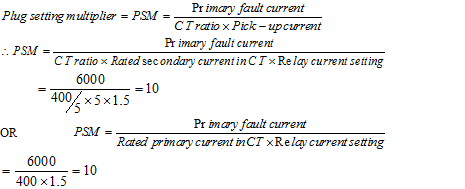Corresponding to PSM of 10, from a given table operating time is 2.8 seconds at TSM = 1.

Now, for TSM = 0.4 -

Therefore,

Actual relay operating time    = Operating time from table x Time setting multiplier

= 2.8 x 0.4 = 1.12 seconds

5.   A 10 A IDMT relay has a current setting of 150 % and has a time multiplier setting of 0.5. The relay is connected in a circuit through a current transformer having ratio 400 : 5 A. Calculate the time of operation of the relay if the circuit carries a fault current of 6000 A. The relay characteristic for TSM = 1 is given as:

 PSM: 2 3.6 5 10 15 20 Operating time in seconds 10 6 3.9 2.8 2.2 2.1

Solution: - Rated relay current = 10 A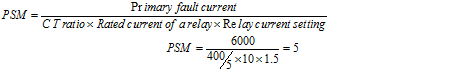Corresponding to PSM of 5, from the given table, operating time is 3.9 seconds at TSM = 1.

Now, for TSM = 0.5 -

Therefore, Actual relay operating time = Operating time x Time setting multiplier

= 3.9 x 0.5 = 1.95 seconds

6. An IDMT over-current relay has a current setting of 150 % and has a time multiplier setting of 0.5. The relay is connected in a circuit through a current transformer having ratio 500 : 5 A. Calculate the time of operation of the relay if the circuit carries a fault current of 6000 A. The relay characteristic for TSM = 1 is given as:

 PSM: 2 4 5 8 10 20 Operating time in seconds 10 5 4 3 2.8 2.4

Solution: - Rated secondary current of C T = 5 ACorresponding to PSM of 8, from a given table operating time is 3 seconds at TSM = 1.

Now, for TSM = 0.5 -

Therefore, Actual relay operating time = Operating time x Time setting multiplier

= 3 x 0.5 = 1.5 seconds

7.   Consider a system: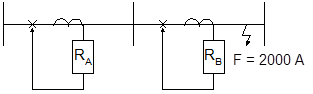Given that: Fault current = 2000 A, CT ratios for each relay = 200 / 1, RB is set at 100 % and RA is set at 125 %.

For discrimination the time grading margin between the relays are 0.5 sec. Determine the time of operation of the two relays and TSM of relay RA. Assuming relay RB has TSM = 0.2. At TSM = 1 operating time at various PSM are:

 PSM: 2 4 5 8 10 20 Operating time in seconds 10 5 4 3.2 2.8 2.4

Solution: -

Part-I: – When Fault is near B, the PSM is –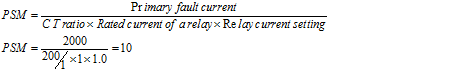Corresponding to PSM of 10, from a given table operating time is 2.8 seconds at TSM of 1.

Now, for TMS = 0.2 -

Therefore, Actual relay operating time  = Operating time x Time setting multiplier

= 2.8 x 0.2 = 0.56 seconds

Part-II: – When Fault is near B, the PSM for relay RA is –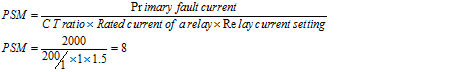Corresponding to PSM of 8, from a given table operating time is 3.2 seconds at TSM = 1.

Now operating time for relay RA    = operating time for relay RB + time grading margin

= 0.56 + 0.5   =      1.06 sec

Therefore, Actual relay RA operating time = Operating time x Time setting multiplier

1.06          = 3.2 x TMS

=> TMS      = 0.33125

8.   Design the time current grading scheme for the following system: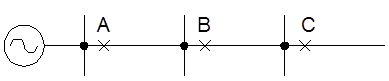Relay Point CT ratio Fault current A 400 / 5 6000 A B 200 / 5 5000 A C 200 / 5 4000 A

At TSM = 1, the time current characteristic of the relay is as given below:

 PSM: 2 3 5 10 15 20 Operating time in seconds 10 6 3.9 3 2.2 2.1

Solution: -

Part-1: - Assume current setting of 100 % and TMS is 0.1

For relay RC -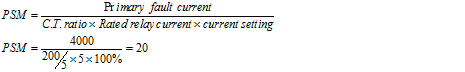Corresponding to PSM of 20, from a given table operating time is 2.1 seconds at TSM = 1.

Now, for TMS = 0.1 -

Therefore, Actual relay operating time    = Operating time x Time setting multiplier

= 2.1 x 0.1 = 0.21 seconds

Now for the same fault current i.e. 4000 A the relay at B must be set to operate as a back-up protection, so assume 0.5 second (time delay step) longer than the relay at C i.e. 0.21 + 0.5 = 0.71 sec

The current setting at B must be increased as compared to that at C to allow for any increase in load caused by load at C, setting the relay current setting at B as 125 %. Thus for 4000 A –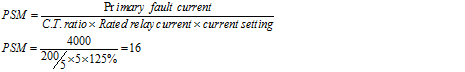Corresponding to PSM of 16, from a given table operating time is 2.2 seconds (i.e. near about same as PSM of 15) at TSM = 1.

Now operating time for relay RB    = operating time for relay RC + time grading margin

= 0.21 + 0.5   =      0.71 sec

Therefore, Actual relay RB operating time = Operating time x Time setting multiplier

0.71     = 2.2 x TMS

=> TMS   = 0.323

Part-2: - Assume current setting of 125 % and TMS calculated is 0.323

When Fault is near B, the PSM is –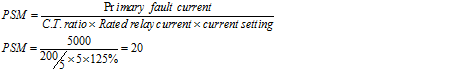Corresponding to PSM of 20, from a given table operating time is 2.1 seconds at TSM = 1.

Now, for TMS = 0.323 -

Therefore, Actual relay operating time    = Operating time x Time setting multiplier

= 2.1 x 0.323 = 0.6783 seconds

Now for the same fault current i.e. 5000 A the relay at A must be set to operate as a back-up protection, so assume 0.5 second (time delay step) longer than the relay at B i.e. 0.6783 + 0.5 = 1.1783 sec

The current setting at A automatically gets increased without increasing the setting of the relay by virtue of its CT ratio. So keeping the relay current setting at A as 125 % i.e. same as that of the relay at B. Thus PSM of relay A, when fault takes place at relay B i.e. for 5000 A –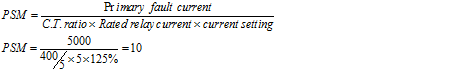Corresponding to PSM of 10, from a given table operating time is 3.0 seconds at TSM = 1.

Now operating time for relay RA    = operating time for relay RB + time grading margin

= 0.6783 + 0.5       =      1.1783 sec

Therefore, Actual relay RA operating time = Operating time x Time setting multiplier

1.1783  = 3.0 x TMS

=> TMS      = 0.393

Part-3: - Assume current setting of 125 % and TMS calculated is 0.393

When Fault is near A, the PSM is -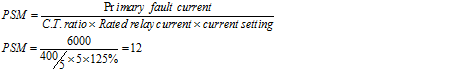Corresponding to PSM of 12, from a given table operating time is 2.6 seconds at TSM = 1.

Now, for TMS = 0.393 -

Therefore, Actual relay operating time    = Operating time x Time setting multiplier

= 2.6 x 0.393 = 1.0218 seconds

9.     Determine the operating time of the relay for the current rating of a relay is 5 ampere having a current setting of 200 % and a time setting multiplier of 0.3 connected to supply circuit through a 400 / 5 current transformer when the circuit carries a fault current of 4000 amp. At TMS = 1, operating time at various PSM are:

 PSM: 2 4 5 8 10 20 Operating time in seconds 10 5 4 3 2.8 2.4

Solution: - Rated secondary current of CT = 5 amp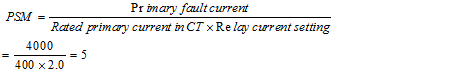Corresponding to PSM of 5, from a given table operating time is 4 seconds at TSM = 1.

Now, for TSM = 0.3 -

Therefore, Actual relay operating time = Operating time x Time setting multiplier

= 4 x 0.3 = 1.2 seconds